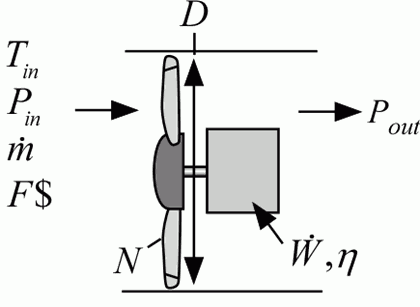Contents - index

Fan1_CLFan1_CL provides a model of a vane axial fan that is based on curves for dimensionless pressure rise (DP/(rho*D^2*N^2)) as a function of dimensionless volumetric flow rate (V_dot/(D^3*N) and dimensionless power (W_dot/(rho*D^5*N^3)).  These curves were generated by collapsing performance data from several manufacturers and models.

Inputs:

F\$:  fluid string identifier

m_dot:  mass flow rate (kg/s)

T_in:  inlet temperature (K, C, F, or R)

P_in:  inlet pressure (bar, atm, Pa, kPa, or MPa)

N:  rotational speed (rev/s)

D:  blade diameter (m or ft)

Outputs:

P_out:  outlet pressure (bar, atm, Pa, kPa, or MPa)

W_dot:  power (W, kW, or Btu)

T_out:  outlet temperature (K, C, F, or R)

eta:  efficiency

Example:

\$UnitSystem SI Mass J K Pa

F\$='Air'

V_dot=10000 [cfm]*convert(cfm,m^3/s)

T_in=300 [K]

P_in=101325 [Pa]

rho=density(Air, P=P_in,T=T_in)

m_dot=V_dot*rho

N=3500 [rev/min]*convert(rev/min, rev/s)

D=21.38 [inch]*convert(inch,m)

Call fan1_cl(F\$, m_dot, T_in, P_in, N, D: P_out, W_dot, T_out, eta)

DP=P_out-P_in

{Solution:

W_dot = 5554 [W] or 7.448 [hp]

DP = 851.5 [Pa] or 3.419 [in H2O]

eta = 0.7236}